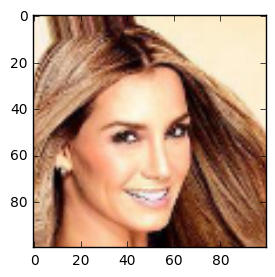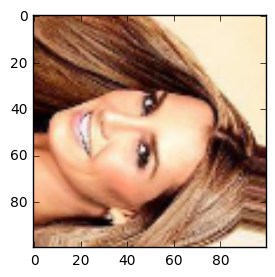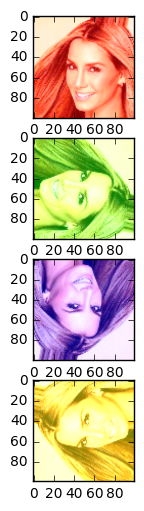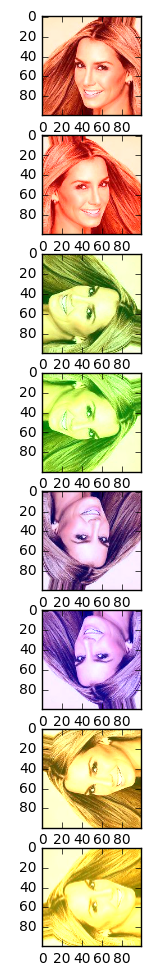## Dihydral Symmetry¶

Tensoflow Implementation of [Die16] Dieleman, Sander, Jeffrey De Fauw, and Koray Kavukcuoglu; "Exploiting cyclic symmetry in convolutional neural networks"

• Cyclic group of order 4: $C_4$
• Dihydral group of order 4 ($C_4$ plus horizontal/vertical flipping): $D_4$

### Equivariance¶

A function $f$ is equivariant to a class of transformations $\mathcal T$ if $$\forall \mathbf T \in \mathcal T: \exists \mathbf T' : f(\mathbf Tx) = \mathbf T'f(x)$$ with a transformation $\mathbf T'$

#### Same-Equivariance¶

A function is same-equivariant if it's equivariant and $\mathbf T = \mathbf T'$.

### Invariance¶

A function $f$ is invariant to a class of transformation $\mathbf T \in \mathcal T$ if $$f(\mathbf Tx) = f(x)$$

In :
%matplotlib inline
import matplotlib.pyplot as plt
import numpy as np
from libs import utils
from skimage.transform import resize

In :
dirname = '/Users/christian/lehre-extern/tensorflow/CADL_my/session-1/img_align_celeba/'

# Load every image file in the provided directory
filenames = [os.path.join(dirname, fname)
for fname in os.listdir(dirname)]

# Make sure we have exactly 100 image files!
filenames = filenames[:100]
assert(len(filenames) == 100)
# Read every filename as an RGB image
imgs = [plt.imread(fname)[..., :3] for fname in filenames]

# Crop every image to a square
imgs = [utils.imcrop_tosquare(img_i) for img_i in imgs]

# Then resize the square image to 100 x 100 pixels
imgs = [resize(img_i, (100, 100)) for img_i in imgs]

imgs = np.array(imgs)#, dtype = 'float32')
#plt.figure(figsize=(3, 3))
#plt.imshow(imgs)
batch_size=10
X = imgs[:batch_size]
X.shape

Out:
(10, 100, 100, 3)

### Operations¶

• Cyclic / Dihydral Slicing
• Cyclic / Dihydral Pooling
• Cyclic / Dihydral Rolling
• Cyclic / Dihydral Stacking

### Cyclic Slicing¶

• Minibatch $X$
$$S(X) = [X, rX, r^2X,r^3X]^T$$

with

• $r$: clockwise rotation by 90 degrees

The size of the minibatch increases (4 times). The rotated examples are stacked across the batch dimension.

### Dihydral Slicing¶

• Minibatch $X$
$$S(X) = [X, mX, rX, rmX, r^2X, r^2mX, r^3X, r^3mX,]^T$$

with

• $r$: clockwise rotation by 90 degrees
• $m$: horizontal flipping

The size of the minibatch increases (8 times). The rotated and/or flipped examples are stacked across the batch dimension.

In :
import tensorflow as tf

In :
g = tf.get_default_graph()
sess = tf.Session(graph=g)

In :
def get_rotations(X, r):
p = len(r)
X_ = [X[i] for i in range(p)]
X_rot = [tf.map_fn(lambda img: tf.image.rot90(img, r[i]), x)
for i, x in enumerate(X_)]
return X_rot

In :
def concat_horizonal_flipped(X):
X_m = tf.reverse(X, [False, False, True, False])
return tf.concat(0, (X, X_m))

In :
X_hflipped = concat_horizonal_flipped(X)
X_r = sess.run(X_hflipped)
plt.figure(figsize=(3, 3))
plt.imshow(X_r)

Out:
<matplotlib.image.AxesImage at 0x1117eba90>In :
def cyclic_slicing(X):
X_ = get_rotations([X]*4, r=(0, 3, 2, 1))
return tf.concat(0, X_)

In :
def dyhydral_slicing(X):
X_ = concat_horizonal_flipped(X)
return cyclic_slicing(X_)

In :
X_sliced_4 = cyclic_slicing(X)
X_s_4 = sess.run(X_sliced_4)

In :
X_sliced_8 = dyhydral_slicing(X)
X_s_8 = sess.run(X_sliced_8)

In :
plt.figure(figsize=(3, 3))
plt.imshow(X_s_8)

Out:
<matplotlib.image.AxesImage at 0x11dfbc588>## Cyclic Pooling¶

Input to pooling layer

$$X = [X_0, X_1, X_2, X_3 ]^T$$$$P(X) = p(X_0, r^{-1}X_1,r^{-2}X_2,r^{-3}X_3)$$

with

• $p$: a permutation invariant pooling operation
• $r^{-1}$: counter-clockwise rotation by 90 degree

Pooling is typically done in the dense layers. So no back rotation (or flipping) is needed.

In :
def dense_pooling(X, pool_op, p=8):
# pool_op has to be a tf reduce operator
# no spatial structure -> no backrotation (r^-1) needed
shape = tf.shape(X)
X_ = tf.reshape(X, (p, shape//p, shape))
return pool_op(X_, (0))

In :
X_s_8_shape = X_s_8.shape
X_s_8_  = X_s_8.reshape((X_s_8_shape, -1))
print (X_s_8_.shape)

(80, 30000)

In :
pool_op = tf.reduce_mean
sess.run(dense_pooling(X_s_8_, pool_op, p=8)).shape

Out:
(10, 30000)

#### Equivariance of the Slicing operator $S$ to rotations $r$¶

$X$ is minibatch

$$S(rX) = [rX, r^2X, r^3X, X]^T = \sigma S(X)$$

with the cyclic permutation $\sigma$ shifting the elements backwards.

In :
def segmentation(X, p=8):
shape = tf.shape(X)
return tf.reshape(X, (p, shape//p, shape, shape, shape))

In :
# just for visualization purpose
shape = np.shape(X)
X = X.copy()
X_ = np.reshape(X, (8, shape//8, shape, shape, shape))
X_[0,:,:,:,0] += 0.3
X_[1,:,:,:,0] += 0.5
X_[2,:,:,:,1] += 0.3
X_[3,:,:,:,1] += 0.5
X_[4,:,:,:,2] += 0.3
X_[5,:,:,:,2] += 0.5
X_[6,:,:,:,0] += 0.3
X_[6,:,:,:,1] += 0.3
X_[7,:,:,:,0] += 0.5
X_[7,:,:,:,1] += 0.5
X_[X_>1.] = 1.
return np.concatenate((X_, X_,X_, X_, X_, X_,X_, X_), 0)

In :
# just for visualization purpose
shape = np.shape(X)
X = X.copy()
X_ = np.reshape(X, (4, shape//4, shape, shape, shape))
X_[0,:,:,:,0] += 0.5
X_[1,:,:,:,1] += 0.5
X_[2,:,:,:,2] += 0.5
X_[3,:,:,:,0] += 0.5
X_[3,:,:,:,1] += 0.5
X_[X_>1.] = 1.
return np.concatenate((X_, X_,X_, X_), 0)

In :
X_colors_4 = add_colors_4(X_s_4)

In :
plt.figure(figsize=(2, 6))
p=4
for i in range(p):
plt.subplot(p, 1, i+1)
plt.imshow(X_colors_4[batch_size*i, :, :, :])In :
X_colors_8 = add_colors_8(X_s_8)

In :
plt.figure(figsize=(5, 12))
p=8
for i in range(p):
plt.subplot(p, 1, i+1)
plt.imshow(X_colors_8[batch_size*i, :, :, :])In :
def cyclic_permutation(X, shift=1, p=8):
perm = np.roll(range(p), shift=-shift)
X_ = segmentation(X, p=p)
X_concat = [X_[perm[i]] for i in range(p)]
return tf.concat(0, X_concat)

In :
X_p = sess.run(cyclic_permutation(X_sliced_4, 2, 8))
X_p.shape

Out:
(40, 100, 100, 3)
In :
plt.figure(figsize=(3, 3))
plt.imshow(X_p)

Out:
<matplotlib.image.AxesImage at 0x121ddd940>### Stacking $T$¶

Stacking along the feature dimensions:

$$T(X) = [X_0, r^{-1}X_1, r^{-2}X_2, r^{-3}X_3]$$

with $X = [X_0, X_1, X_2, X_3]^T$

In :
def stack(X):
X_ = segmentation(X, 4)
X_ = [X_[i] for i in range(4)]
r = (0, 1, 2, 3)
X_ = get_rotations(X_, r=r)
X_concat = [X_[i] for i in range(4)]
return tf.concat(3, X_concat)

In :
X_stacked_4 = sess.run(stack(cyclic_permutation(X_colors_4, 3, p=4)))
X_stacked_4.shape

Out:
(10, 100, 100, 12)
In :
plt.figure(figsize=(6, 2.5))
p=4
for i in range(p):
plt.subplot(1, p, i+1)
plt.imshow(X_stacked_4[0, :, :, (i*3):(i*3)+3])